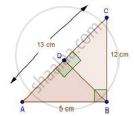Share

Books Shortlist
Your shortlist is empty

# Solution for A Triangle Has Sides 5 Cm, 12 Cm and 13 Cm. Find the Length to One Decimal Place, of the Perpendicular from the Opposite Vertex to the Side Whose Length is 13 Cm. - CBSE Class 10 - Mathematics

ConceptApplication of Pythagoras Theorem in Acute Angle and Obtuse Angle

#### Question

A triangle has sides 5 cm, 12 cm and 13 cm. Find the length to one decimal place, of the perpendicular from the opposite vertex to the side whose length is 13 cm.

#### SolutionLet, AB = 5cm, BC = 12 cm and AC = 13 cm. Then, AC2 = AB2 + BC2. This proves that ΔABC is a right triangle, right angles at B. Let BD be the length of perpendicular from B on AC.

Now, Area ΔABC =1/2(BCxxBA)

=1/2(12xx5)=30" cm"^2

Also, Area of ΔABC =1/2ACxxBD=1/2(13xxBD)

rArrBD=60/13cm

Is there an error in this question or solution?

#### Video TutorialsVIEW ALL 

Solution for question: A Triangle Has Sides 5 Cm, 12 Cm and 13 Cm. Find the Length to One Decimal Place, of the Perpendicular from the Opposite Vertex to the Side Whose Length is 13 Cm. concept: Application of Pythagoras Theorem in Acute Angle and Obtuse Angle. For the course CBSE
S